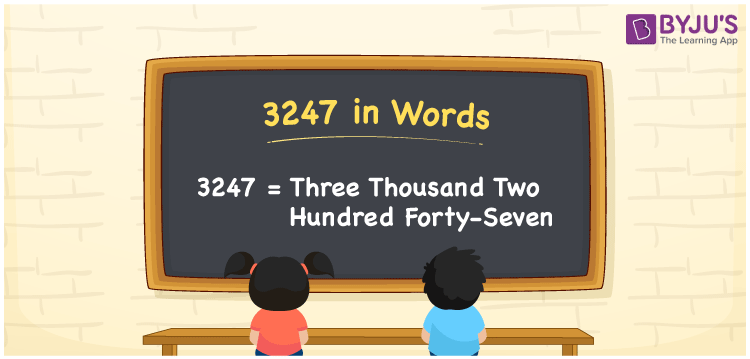# 3247 in Words

3247 in words is Three thousand two hundred forty-seven. If you saved Rs. 3247 in 4 months, it can be written as “I saved Rs. Three thousand two hundred forty-seven in four months”. Also, 3247 is also a cardinal number. In this article, let us learn the method of writing 3247 in words using the place value system in detail.

 3247 in Words: Three Thousand Two Hundred Forty-seven. Three Thousand Two Hundred Forty-seven in Numerical Form: 3247.

## 3247 in English Words## How to Write 3247 in Words?

The place values of the numeral 3247 are presented below:

 Thousands Hundreds Tens Ones 3 2 4 7

The expanded form of 3247 is as follows:

= 3 × Thousand + 2 × Hundred + 4 × Ten + 7 × One

= 3 × 1000 + 2× 100 + 4 × 10 + 7 × 1

= 3000 + 200 + 40 + 7

= 3247

= Three thousand two hundred forty-seven

Hence, 3247 in words is three thousand two hundred forty-seven.

3247 in words – Three thousand two hundred forty-seven

Is 3247 an odd number? – Yes

Is 3247 an even number? – No

Is 3247 a perfect square number? – No

Is 3247 a perfect cube number? – No

Is 3247 a prime number? – No

Is 3247 a composite number? – Yes

## Frequently Asked Questions on 3247 in Words

### How to spell 3247 in English words?

3247 in words is three thousand two hundred forty-seven.

### Simplify 3200 + 47, and express it in words.

Simplifying 3200 + 47, we get 3247. Hence, 3247 in words is three thousand two hundred forty-seven.

### How to write three thousand two hundred forty-seven in numbers?

Three thousand two hundred forty-seven in numbers is 3247.